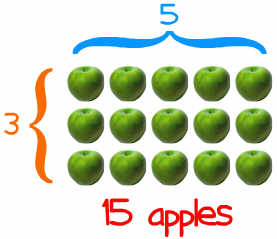Definition of

# MultiplyThe basic idea of multiplying is repeated addition.

Example: 5 × 3 = 5 + 5 + 5 = 15

But as well as multiplying by whole numbers, we can also multiply by fractions, decimals and more.

Example: 5 × 3½ = 5 + 5 + 5 + (half of 5) = 17.5

Try dragging the numerals to the blue boxes below: